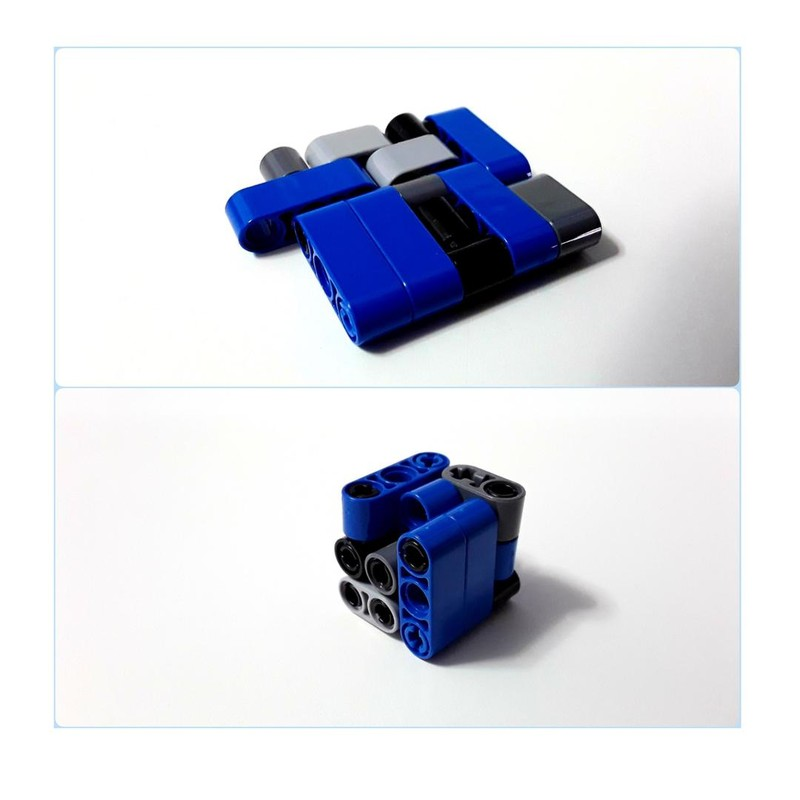### Puzzle 26

•## 322

### LIKES

Puzzle 26
No, this is not my 26th puzzle. It is just my second - but do visit the Quiangle Puzzle for more thrills ;)
Summary: 26 is a special number - It stands between a square (25) and a cube (27). The instructions tell you how to setup the puzzle in a 5x5 square with one extra 1x1 square (surface area 26). The puzzle is how to fold it into a 3x3x3 cube - with one 1x1x1 cube missing (volume 26).
The puzzle therefore demonstrates this property of 26, but it doesn't prove the much more interesting property: 26 is the only number  (to be clear, we refer only to natural numbers, not numbers with decimal points) to be stuck this way between a square and a cube. The proof for that is remarkably complicated and requires advanced algebra.
For some background. if you got this far in the description ;) A number x is a square if you can arrange x dots in a square. 4 is a square

*  *
*  *

A number x is a cube if you can arrange x cubes to form a larger cube. 8 is a cube: stack two of the 2x2 squares on top of each other - or count the corners of dice.

Many numbers have interesting properties, such as "smallest\largest number that..." but it is not often that a number is one-of-a-kind, and 26 is such a one.

If you enjoy math-related content, press the MATH tag on the side, and see more such models from me - free of charge :)

Color note - I made everything [no color\any color] because the colors don't matter - it's math. The instructions and pictures follow the colors I had at home.